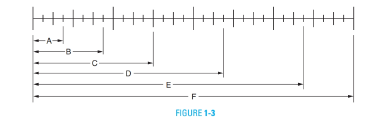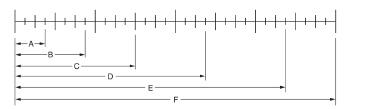# Write the fractional part that each length, A through F, represents of the total shown on the scale in Figure 1-3. A = . B = . C = . D = . E = . F = .### Mathematics for Machine Technology

7th Edition
John C. Peterson + 1 other
Publisher: Cengage Learning
ISBN: 9781133281450

#### Solutions

Chapter
Section### Mathematics for Machine Technology

7th Edition
John C. Peterson + 1 other
Publisher: Cengage Learning
ISBN: 9781133281450
Chapter 1, Problem 1A
Textbook Problem
33 views

## Write the fractional part that each length, A through F, represents of the total shown on the scale in Figure 1-3.A = .B = .C = .D = .E = .F = .

To determine

Evaluate the fractional part length of A, B, C, D, E and F.

The fractional part length of A, B, C, D, E and F are 332, 732, 38, 1932, 2732 and 1 respectively.

### Explanation of Solution

Given:

All the dimensions are shown in below Fig:Concept used:

Fraction of the each part can determine with given expression.

Fraction=nN   .... (1)

Here, length of each part is n and total length is N.

Calculation:

From below Fig:Fraction A is calculated as:

Substitute 3 for n and 32 for N.

A=332

Fraction B is calculated as:

Substitute 7 for n and 32 for N.

B =732

Fraction C is calculated as:

Substitute 12 for n and 32 for N.

C =1232=38

Fraction D is calculated as:

Substitute 19 for n and 32 for N.

D =1932

Fraction E is calculated as:

Substitute 27 for n and 32 for N.

E =2732

Fraction F is calculated as:

Substitute 32 for n and 32 for N.

F =3232F =1

Thus, the fractional part length of A, B, C, D, E and F are 332, 732, 38, 1932, 2732 and 1 respectively.

Conclusion:

The fractional part length of A, B, C, D, E and F are 332, 732, 38, 1932, 2732 and 1 respectively.

#### The Solution to Your Study Problems

Bartleby provides explanations to thousands of textbook problems written by our experts, many with advanced degrees!

Get Started

Find more solutions based on key concepts
For y=43+2x, y = _____. a) 4(3 + 2x)3/2 b) 4(3 + 2x)3/2 c) 2(3 + 2x)1/2 d) 2(3 + 2x)1/2

Study Guide for Stewart's Single Variable Calculus: Early Transcendentals, 8th

In Exercises 31-34, evaluate h(2), where h = g f. 31. f(x) = x2 + x + 1; g(x) = x2

Applied Calculus for the Managerial, Life, and Social Sciences: A Brief Approach

In Exercises 5-8, graph the given function or equation. 2x3y=12

Finite Mathematics and Applied Calculus (MindTap Course List)

Self Check :Evaluate using Constant Property: k=1612.

College Algebra (MindTap Course List)

Define extraneous variable and explain how extraneous variables can become confounding variables.

Research Methods for the Behavioral Sciences (MindTap Course List)

ReminderRound all answers to two decimal places unless otherwise indicated. River FlowThe graph in Figure 1.37 ...

Functions and Change: A Modeling Approach to College Algebra (MindTap Course List)